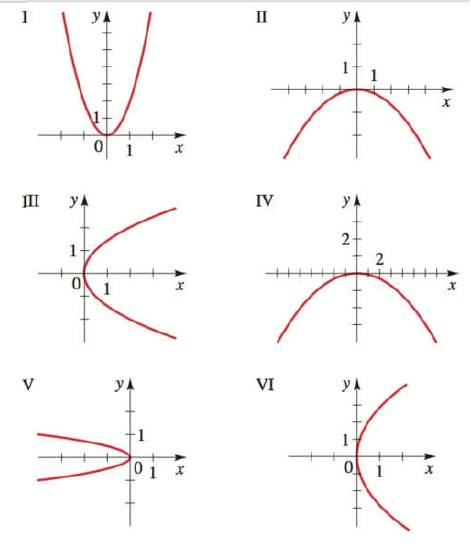Chapter 12.1, Problem 10E### Algebra and Trigonometry (MindTap ...

4th Edition
James Stewart + 2 others
ISBN: 9781305071742

#### Solutions

Chapter
Section### Algebra and Trigonometry (MindTap ...

4th Edition
James Stewart + 2 others
ISBN: 9781305071742
Textbook Problem

# 5-10 Graphs of Parabolas Match the equation with the graphs labeled I-IV. Give reasons for your answers. 12 y + x 2 = 0To determine

To match:

The equation with the graph 12y+x2=0 and give the reason.

Explanation

Given:

The given graphs are shown below.

Figure (1)

Approach:

The equation of parabola is given by,

x2=4py

And, the properties for the parabola are as follows,

Vertex =V(0,0).

Focus =F(0,p).

Directrix y=p.

The parabola opens downwards if p<0 or to the upwards if p>0.

Calculation:

Consider the given equation.

12y+x2=0x2=12y

Compare the above equation with the equation of parabola.

### Still sussing out bartleby?

Check out a sample textbook solution.

See a sample solution

#### The Solution to Your Study Problems

Bartleby provides explanations to thousands of textbook problems written by our experts, many with advanced degrees!

Get Started